### 上海大众 途安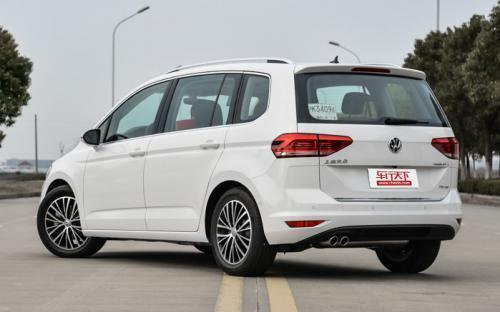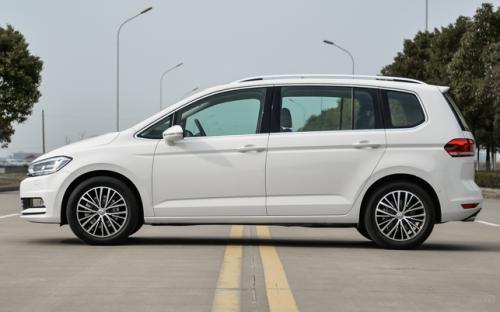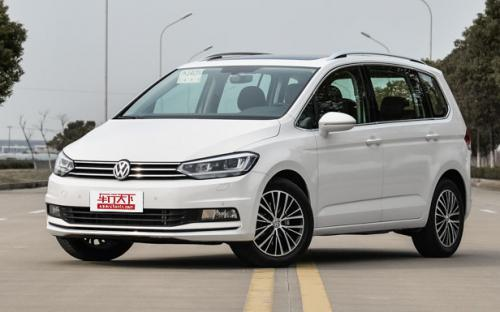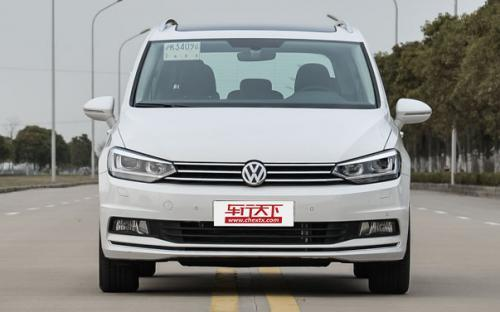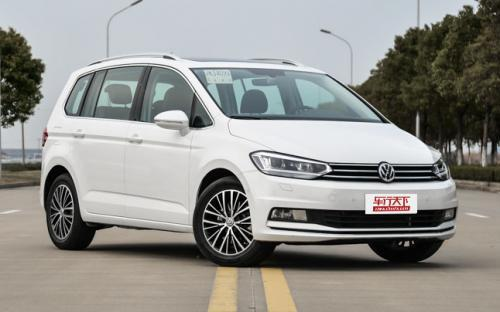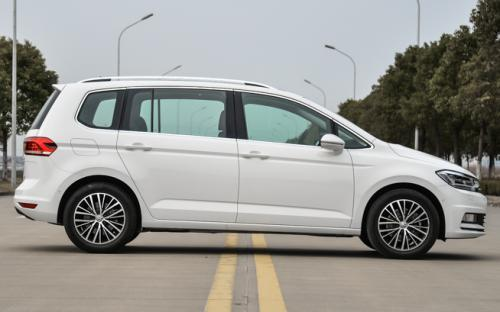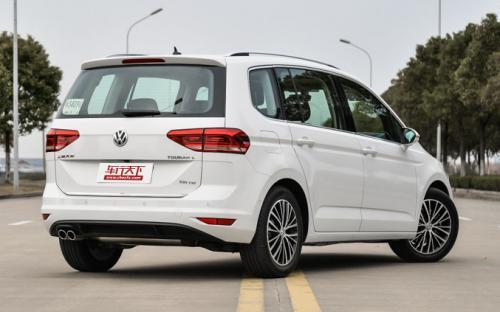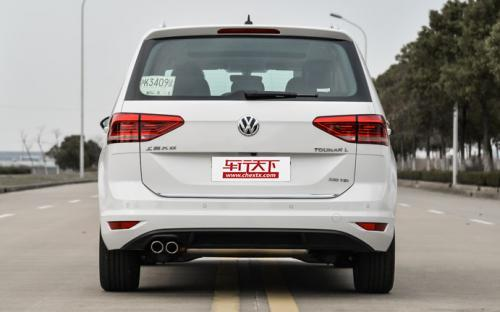7 种颜色可选2016款最低售价：15.58 万元起

4527(mm)1829(mm)1659(mm)##### 配置亮点：
• 胎压监测装置

• ISOFIX儿童座椅接口

• 车身稳定控制(ESC/ESP/DSC等)

• 电动天窗

• 定速巡航

• 后倒车雷达

• 真皮座椅

• GPS导航系统

• 氙气大灯

• 后视镜加热

• 提交
2016款 280TSI DSG豪华版 (139张)
• 2016款 280TSI DSG豪华版 (139张)
• 2016款 330TSI DSG豪华版 (178张)
• 2016款 280TSI 手动舒适版 (117张)
• 上海大众 途安 绕车实拍• 上海大众 途安 在售车型

排量 车型 厂商指导价 本地最低报价 购车工具
1.4T
15.58万
15.58万

280TSI DSG风尚版 7挡双离合
16.98万
17.28万
17.28万

280TSI DSG舒适版 7挡双离合
18.68万
280TSI DSG舒雅版 7挡双离合
19.98万
280TSI DSG豪华版 7挡双离合
21.98万
330TSI DSG豪华版 7挡双离合
23.08万
23.08万

1.8T
15.58万
15.58万

280TSI DSG风尚版 7挡双离合
16.98万
17.28万
17.28万

280TSI DSG舒适版 7挡双离合
18.68万
280TSI DSG舒雅版 7挡双离合
19.98万
280TSI DSG豪华版 7挡双离合
21.98万
330TSI DSG豪华版 7挡双离合
23.08万
23.08万

上海大众 途安 经销商

查看更多 >>

### 上海大众 途安 动力加速

途安 0-100公里加速时间分布在 8.5-11秒 属于 运动级

动力级别 加速时间 车型
运动级(2款)
9.0s
280TSI 手动风尚版280TSI 手动舒适版
8.5s
330TSI DSG豪华版
民用级(2款)
9.3s
280TSI DSG风尚版280TSI DSG舒适版280TSI DSG舒雅版280TSI DSG豪华版
11.0s
1.4T 手动风尚版5座1.4T 手动舒适版5座1.4T DSG舒适版5座1.4T 手动豪华版5座1.4T DSG豪华版5座1.4T DSG旗舰版5座

上海大众 途安 视频

上海大众 途安 新闻资讯

# 上汽大众途安L上市 售价15.58-23.08万元

新闻 超过9122次关注

2016年3月18日，上汽大众在途安L的诞生地长沙正式公布了新车的上市价格，新车搭载了1.4TSI和1.8TSI两款动力总成共计7款车型，售价区间为15.58-23.08万元。

# 大众召回新宝来 速腾 高尔夫 途安 途观

碰撞安全 超过9271次关注

日前，一汽-大众汽车有限公司、上海大众汽车有限公司根据《缺陷汽车产品召回管理条例》的要求，向国家质检总局备案了召回计划，决定自即日起，召回新宝来、速腾、高...

# 2015款途安1.4T风尚版实拍 解读低配车

导购 超过3607次关注

15万元左右的价格上如果要选择一款适合家用的合资品牌MPV，日系产品依旧占据了大半市场，外形漂亮、配置丰富、内部空间布局合理是日系MPV的特点。可确实又有不少消...

# 车展体验2013款新途安 请叫我小年轻！

导购 超过3444次关注

本次广州车展，上海大众带来了2013款途安，为了吸引更多年轻人的注意力，厂家为其增加了全新的车身和内饰颜色，第一眼看上的确活力了不少。再配上新样式的轮毂和大...

# 测试途安1.4T自动挡 注重燃油经济性

评测 超过3730次关注

在大众的国内产品序列中，途安一直属于表现比较冷淡的一款，它在技术上的换代也属于节奏比较慢的。现如今，2011款途安换装了1.4TSI发动机，我们之前还一直没有对它...

# 上海大众7款纪念版于2014成都车展上市

新闻 超过2517次关注

在本届成都车展上，上海大众宣布向市场推出7款30周年纪念版车型，分别是30周年纪念版的POLO、桑塔纳、朗逸、朗行、帕萨特、途观、途安，它们的价格区间覆盖11.88-25...

# 上海大众2015款途安上市 售14.98万起

新闻 超过2624次关注

近日上海大众宣布，正式向市场推出2015款途安，新车主要增添了内外配色选择，同时对配置进行了调整，6款车型的价格区间为14.98-20.68万元。另外此次上市车型均为5座...

# 上海大众途安1.6L申报图 或年内上市

新闻 超过2239次关注

日前，我们收到了一组上海大众途安1.6L车型的申报图，新车或将在今年下半年正式上市。

猜你喜欢

﻿
• 快速找车
• 选择品牌
• 选择品牌
• A  奥迪
• A  阿斯顿·马丁
• A  阿尔法·罗密欧
• B  宝沃
• B  布加迪
• B  巴博斯
• B  保时捷
• B  宾利
• B  奔驰
• B  宝马
• B  本田
• B  别克
• B  标致
• B  比亚迪
• B  宝骏
• B  北汽制造
• B  北汽新能源
• B  北汽幻速
• B  北汽威旺
• B  北京汽车
• B  奔腾
• B  北汽绅宝
• C  长安
• C  长安商用
• C  长城
• C  昌河
• D  大众
• D  道奇
• D  DS
• D  东南
• D  东风风神
• D  东风风行
• D  东风小康
• D  东风风度
• D  东风
• F  福特
• F  丰田
• F  菲亚特
• F  法拉利
• F  福田
• F  福迪
• F  福汽启腾
• G  观致
• G  广汽传祺
• G  广汽吉奥
• G  GMC
• H  红旗
• H  汉腾汽车
• H  哈弗
• H  哈飞
• H  海格
• H  海马
• H  华颂
• H  黄海
• H  华泰
• H  恒天
• J  吉利汽车
• J  捷豹
• J  Jeep
• J  江淮
• J  江铃
• J  金杯
• J  九龙
• J  金旅
• K  凯翼
• K  凯迪拉克
• K  克莱斯勒
• K  科尼塞克
• K  卡威
• K  开瑞
• L  路虎
• L  林肯
• L  劳斯莱斯
• L  兰博基尼
• L  雷克萨斯
• L  铃木
• L  雷诺
• L  理念
• L  力帆
• L  莲花汽车
• L  猎豹
• L  路特斯
• L  陆风
• M  马自达
• M  MG
• M  MINI
• M  玛莎拉蒂
• M  摩根
• M  迈凯轮
• N  纳智捷
• O  欧宝
• O  讴歌
• O  欧朗
• Q  奇瑞
• Q  起亚
• Q  启辰
• R  日产
• R  荣威
• R  瑞麒
• S  三菱
• S  斯威汽车
• S  萨博
• S  smart
• S  斯柯达
• S  斯巴鲁
• S  思铭
• S  双龙
• S  上汽大通
• S  双环
• T  特斯拉
• T  腾势
• W  沃尔沃
• W  五菱汽车
• W  五十铃
• W  威兹曼
• W  威麟
• X  现代
• X  雪佛兰
• X  雪铁龙
• X  西雅特
• Y  一汽
• Y  英菲尼迪
• Y  英致
• Y  依维柯
• Y  野马汽车
• Y  永源
• Z  众泰
• Z  中华
• Z  中兴
• Z  知豆
• 选择车系
• 选择车系
• 车型对比
• 选择品牌
• 选择品牌
• A  奥迪
• A  阿斯顿·马丁
• A  阿尔法·罗密欧
• B  宝沃
• B  布加迪
• B  巴博斯
• B  保时捷
• B  宾利
• B  奔驰
• B  宝马
• B  本田
• B  别克
• B  标致
• B  比亚迪
• B  宝骏
• B  北汽制造
• B  北汽新能源
• B  北汽幻速
• B  北汽威旺
• B  北京汽车
• B  奔腾
• B  北汽绅宝
• C  长安
• C  长安商用
• C  长城
• C  昌河
• D  大众
• D  道奇
• D  DS
• D  东南
• D  东风风神
• D  东风风行
• D  东风小康
• D  东风风度
• D  东风
• F  福特
• F  丰田
• F  菲亚特
• F  法拉利
• F  福田
• F  福迪
• F  福汽启腾
• G  观致
• G  广汽传祺
• G  广汽吉奥
• G  GMC
• H  红旗
• H  汉腾汽车
• H  哈弗
• H  哈飞
• H  海格
• H  海马
• H  华颂
• H  黄海
• H  华泰
• H  恒天
• J  吉利汽车
• J  捷豹
• J  Jeep
• J  江淮
• J  江铃
• J  金杯
• J  九龙
• J  金旅
• K  凯翼
• K  凯迪拉克
• K  克莱斯勒
• K  科尼塞克
• K  卡威
• K  开瑞
• L  路虎
• L  林肯
• L  劳斯莱斯
• L  兰博基尼
• L  雷克萨斯
• L  铃木
• L  雷诺
• L  理念
• L  力帆
• L  莲花汽车
• L  猎豹
• L  路特斯
• L  陆风
• M  马自达
• M  MG
• M  MINI
• M  玛莎拉蒂
• M  摩根
• M  迈凯轮
• N  纳智捷
• O  欧宝
• O  讴歌
• O  欧朗
• Q  奇瑞
• Q  起亚
• Q  启辰
• R  日产
• R  荣威
• R  瑞麒
• S  三菱
• S  斯威汽车
• S  萨博
• S  smart
• S  斯柯达
• S  斯巴鲁
• S  思铭
• S  双龙
• S  上汽大通
• S  双环
• T  特斯拉
• T  腾势
• W  沃尔沃
• W  五菱汽车
• W  五十铃
• W  威兹曼
• W  威麟
• X  现代
• X  雪佛兰
• X  雪铁龙
• X  西雅特
• Y  一汽
• Y  英菲尼迪
• Y  英致
• Y  依维柯
• Y  野马汽车
• Y  永源
• Z  众泰
• Z  中华
• Z  中兴
• Z  知豆
• 选择车系
• 选择车系
• 选择车型
• 选择车型
• 意见反馈